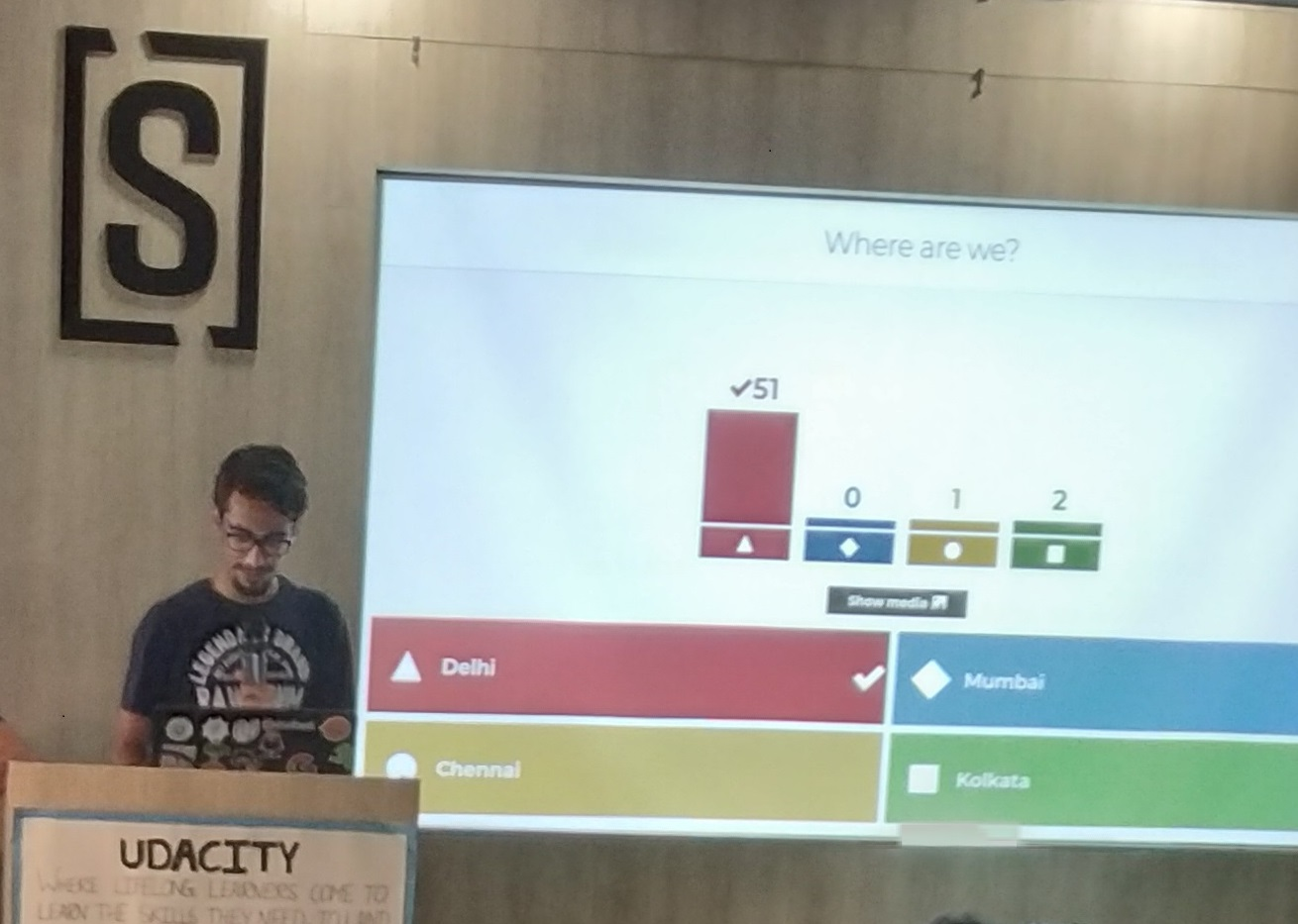# Passing and Returning Objects in C++

In C++ we can pass class’s objects as arguments and also return them from a function the same way we pass and return other variables. No special keyword or header file is required to do so.

### Passing an Object as argument

To pass an object as an argument we write the object name as the argument while calling the function the same way we do it for other variables.
Syntax:

```function_name(object_name);
```

Example: In this Example there is a class which has an integer variable ‘a’ and a function ‘add’ which takes an object as argument. The function is called by one object and takes another as an argument. Inside the function, the integer value of the argument object is added to that on which the ‘add’ function is called. In this method, we can pass objects as an argument and alter them.

## CPP

 `// C++ program to show passing` `// of objects to a function`   `#include ` `using` `namespace` `std;`   `class` `Example {` `public``:` `    ``int` `a;`   `    ``// This function will take` `    ``// an object as an argument` `    ``void` `add(Example E)` `    ``{` `        ``a = a + E.a;` `    ``}` `};`   `// Driver Code` `int` `main()` `{`   `    ``// Create objects` `    ``Example E1, E2;`   `    ``// Values are initialized for both objects` `    ``E1.a = 50;` `    ``E2.a = 100;`   `    ``cout << ``"Initial Values \n"``;` `    ``cout << ``"Value of object 1: "` `<< E1.a` `         ``<< ``"\n& object 2: "` `<< E2.a` `         ``<< ``"\n\n"``;`   `    ``// Passing object as an argument` `    ``// to function add()` `    ``E2.add(E1);`   `    ``// Changed values after passing` `    ``// object as argument` `    ``cout << ``"New values \n"``;` `    ``cout << ``"Value of object 1: "` `<< E1.a` `         ``<< ``"\n& object 2: "` `<< E2.a` `         ``<< ``"\n\n"``;`   `    ``return` `0;` `}`

Output:

```Initial Values
Value of object 1: 50
& object 2: 100

New values
Value of object 1: 50
& object 2: 150

```

### Returning Object as argument

Syntax:

```object = return object_name;
```

Example: In the above example we can see that the add function does not return any value since its return-type is void. In the following program the add function returns an object of type ‘Example'(i.e., class name) whose value is stored in E3.
In this example, we can see both the things that are how we can pass the objects as well as return them. When the object E3 calls the add function it passes the other two objects namely E1 & E2 as arguments. Inside the function, another object is declared which calculates the sum of all the three variables and returns it to E3.
This code and the above code is almost the same, the only difference is that this time the add function returns an object whose value is stored in another object of the same class ‘Example’ E3. Here the value of E1 is displayed by object1, the value of E2 by object2 and value of E3 by object3.

## CPP

 `// C++ program to show passing` `// of objects to a function`   `#include ` `using` `namespace` `std;`   `class` `Example {` `public``:` `    ``int` `a;`   `    ``// This function will take` `    ``// object as arguments and` `    ``// return object` `    ``Example add(Example Ea, Example Eb)` `    ``{` `        ``Example Ec;` `        ``Ec.a = 50;` `        ``Ec.a = Ec.a + Ea.a + Eb.a;`   `        ``// returning the object` `        ``return` `Ec;` `    ``}` `};` `int` `main()` `{` `    ``Example E1, E2, E3;`   `    ``// Values are initialized` `    ``// for both objects` `    ``E1.a = 50;` `    ``E2.a = 100;` `    ``E3.a = 0;`   `    ``cout << ``"Initial Values \n"``;` `    ``cout << ``"Value of object 1: "` `<< E1.a` `         ``<< ``", \nobject 2: "` `<< E2.a` `         ``<< ``", \nobject 3: "` `<< E3.a` `         ``<< ``"\n"``;`   `    ``// Passing object as an argument` `    ``// to function add()` `    ``E3 = E3.add(E1, E2);`   `    ``// Changed values after` `    ``// passing object as an argument` `    ``cout << ``"New values \n"``;` `    ``cout << ``"Value of object 1: "` `<< E1.a` `         ``<< ``", \nobject 2: "` `<< E2.a` `         ``<< ``", \nobject 3: "` `<< E3.a` `         ``<< ``"\n"``;`   `    ``return` `0;` `}`

Output:

```Initial Values
Value of object 1: 50,
object 2: 100,
object 3: 0

New values
Value of object 1: 50,
object 2: 100,
object 3: 200

```

Rated as one of the most sought after skills in the industry, own the basics of coding with our C++ STL Course and master the very concepts by intense problem-solving.

My Personal Notes arrow_drop_upCheck out this Author's contributed articles.

If you like GeeksforGeeks and would like to contribute, you can also write an article using contribute.geeksforgeeks.org or mail your article to contribute@geeksforgeeks.org. See your article appearing on the GeeksforGeeks main page and help other Geeks.

Please Improve this article if you find anything incorrect by clicking on the "Improve Article" button below.

Improved By : aniket173000

Article Tags :
Practice Tags :

5

Please write to us at contribute@geeksforgeeks.org to report any issue with the above content.